Degrading the Effect of Channel Impairment on STBC-OFDM System in Rayleigh Fading Channel | OMICS International
International Journal of Sensor Networks and Data Communications

# Degrading the Effect of Channel Impairment on STBC-OFDM System in Rayleigh Fading Channel

Sinha H1*, Meshram MR2 and Sinha GR1

1Shri Shankaracharya Technical Campus Bhilai, Chhattisgarh, India

2Department of Electronics and Telecommunication Engineering, Government Engineering College, Jagdalpur, Chhattisgarh, India

*Corresponding Author:
Sinha H
PhD Scholar, Shri Shankaracharya Technical Campus
Bhilai, Chhattisgarh
India
Tel: 0788 229 1607
E-mail: [email protected]

Received date: July 06, 2016; Accepted date: August 26, 2016; Published date: August 30, 2016

Citation: Sinha H, Meshram MR, Sinha GR (2016) Degrading the Effect of Channel Impairment on STBC-OFDM System in Rayleigh Fading Channel. Int J Sens Netw Data Commun 5:146. doi: 10.4172/2090-4886.1000146

Copyright: © 2016 Sinha H, et al. This is an open-access article distributed under the terms of the Creative Commons Attribution License, which permits unrestricted use, distribution, and reproduction in any medium, provided the original author and source are credited.

Visit for more related articles at International Journal of Sensor Networks and Data Communications

#### Abstract

This paper indicates the space time block code provides transmit diversity in wireless fading channel during the STBC-OFDM system. OFDM is enforced in broadband wireless access systems as the way to beat wireless channel impairments and to enhance and breadth potency. This research is to analyze the performance of non STBC-OFDM system and STBC-OFDM system. The performance has been evaluated for construction modulation (QAM) technique for orthogonal frequency division multiplexing (OFDM) systems using multiple transmit diversity antenna system in the channel. Conjointly the equation of SNR and BER has been derived for 16-QAM for multiple transmit and receive antenna for STBC-OFDM system by considering the have an effect on of Inter-symbol interference (ISI), phase noise and temporal arrangement noise in Raleigh fading channel.

#### Keywords

STBC; OFDM; Phase noise

#### Introduction

Physical limitations of the wireless medium produce a technical challenge for reliable wireless communication techniques that improve spectral potency and to attain high information rates with low information measure. To overcome varied channel impairments cherish signal attenuation, interference, section noise, temporal order interference have made a colossal contribution to the expansion of wireless communications . Multiple-input multiple-output (MIMO) primarily based communication systems area unit capable accomplishing these objectives, the OFDM system carries the message information on orthogonal subcarriers for parallel transmission; it's wide for its information measure potency used combating the distortion caused by the frequency selective channel or equivalently, the inter-symbol interference in the multi-path attenuation channel . However, the advantage of the OFDM is effectively helpful only when the orthogonality is maintained between sub carriers because of orthogonal characteristics additional information can be transmitted at a precise quantity of band breadth as compare to the opposite technique. Other way to increase diversity gain by mistreatment area time blocks writing STBC technique . The combination of the OFDM with STC with multi path attenuation channel accomplish high spectral potency and high data rate STBC-OFDM will increase the antenna diversity gain [3-5]. This paper derived analytically SNR and BER performance in multiple antenna system. just in case the orthogonality isn't sufficiently invulnerable by any means, its performance could also be degraded thanks to inter-symbol interference (ISI) and inter-channel interference (ICI), temporal order jitter . Obtaining the results of temporal order interference, inter-symbol interference (ISI) problems in OFDM systems. Fading describes the variation of the native channel h (t) due to the varied phases and amplitudes of scatterers. Once the freelance scatter ns is massive and every one scattered contributions area unit noncoherent (not LOS) and of roughly equal energy, then by the central limit theorem h (t) could be a complicated Gaussian variable with zero mean and independent quadrature components. In this paper to analysis non-STBC OFDM system and other part shows the analysis of STBC-OFDM. At the end of the research analysis to determine the equation for Signal to Noise Ratio (SNR) and Bit Error Rate (BER) have been derived analytically using four transmitting antennas and one receiving antenna.

#### Orthogonal Frequency Division Multiplexing (OFDM)

Orthogonal Frequency Division Multiplexing (OFDM) might be a competent technique to perform multicarrier modulation with mainly utilization of bandwidth and high performance characteristics profile against multipath fading channel. At the instant time Orthogonal Frequency Division Multiplexing is broad used for its bandwidth efficiency property credit to its orthogonal characteristic additional information are often transmitted at the certain amount of bandwidth as compare to the other systems. The affects Inter Symbol Interference (ISI) is in addition very low compare to the different type of multiplexing techniques. OFDM has been adopted and enforced in wire and wireless communication system. Unfortunately OFDM is extremely sensitive to the synchronization errors like Carrier frequency offset (CFO), Inter Symbol interference (ISI), and timing jitter . Orthogonality take into account the time-limited advanced exponential signals that represent the various subcarriers at fk=k/Tsym within the OFDM signal, wherever 0 ≤ t ≤ Tsym. These signals clearly define to be orthogonal if the integral of the products for its common (fundamental) periodic zero, the Equation (1) given below,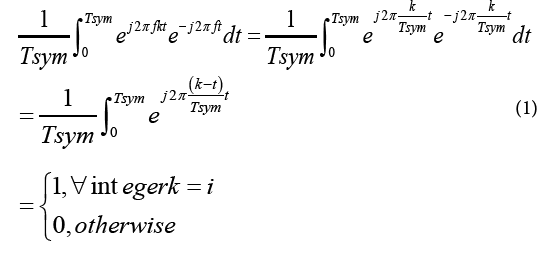Taking the discrete samples with the sampling instances at t ¼ nTs ¼ nTsym=N, n ¼ 0; 1; 2; ---; N_1, Equation (2) can be written in the discrete time domain as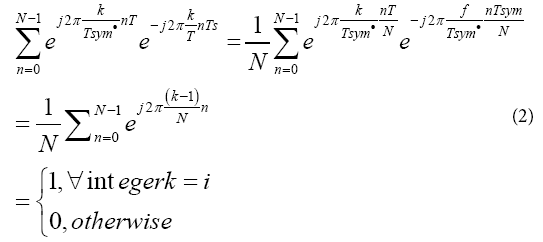The above orthogonality equation is an essential condition for the OFDM signal to be ICI-free. OFDM is extremely sensitive to the synchronization errors like Carrier frequency offset (CFO), timing jitter. The Carrier frequency offset CFO arise primarily due to Doppler Effect, the result is caused by the CFO decrease the signal amplitude and makes interference between the carriers . Timing error would occur either once the clock signal is not properly recovered or once sampling circuit imperfect. Propagation delay of the ICI causes timing error. Other term is AWGN is introduced within the channel through that information is transmitted. The aim of this paper is to analysis the signal to noise ratio (SNR) and by changing the CFO in timing jitter . Analysis has been done and the graphs have been plotted with the assistance of MATLAB Program and other graph also for Bit Error Rate (BER) in keeping with totally different SNR.

Phase noise

Phase noise is rapidly short term, time-varying and random fluctuations that effects on the phase of a signal waveform caused by time domain instabilities occurs in early stages of receiver part, especially in demodulation stage. Presence of phase noise is increased errors for overall system. Therefore this term must be eliminated in order to enhance the error performance.

Timing jitter

In the sampling circuit at the receiver additional error may occur in the determination of the best sampling phase. This means that the sampling instants are non-ideal and is given by- tn = nT + ξn ; Where ξn is the timing jitter of the nth sampling instant normalized by the symbol period T. Elective measurement of phase noise, but in the timedomain associated with actuations in the times of zero-crossings of a phase error.

CFO

In order to suppress the ICI and thereby reduce SNR degradation, the residual CFO must be sufficiently small. For example, when using the 64QAM constellation, it is better to keep the residual CFO below O. OI/s to ensure that SNR < 0.3 dB for moderate SNR. The absolute value of CFO is fε, is either an integer multiple or a fraction of Δf. Now if the fε is normalized to the sub carrier spacing Δf then normalized CFO of the channel is expressed as Where δ is an integer and |?| ≤ 0.5 If the CFO occurs then the symbol transmitted on a certain sub carrier k, will shift to another sub carrier ks = k + δ.

The transmitted OFDM signal for the month symbol is given by the N point complex modulation sequence is given by Equation (3),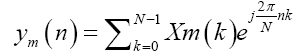(3)

Where n ranges from 0 to N+Ng-1 After passing through a Rayleigh fading channel and LO, the received signal impaired by AWGN and PN can be modeled as;

Where,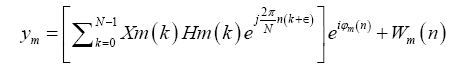(4)

Or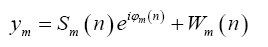(5)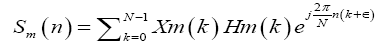(6)

Here Hm(k) is the transfer function of the Rayleigh fading channel at the frequency of the kth carrier and Wm(n) is the complex envelope of the AWGN with zero mean and variance σ2. SNR Equation (7) is given below,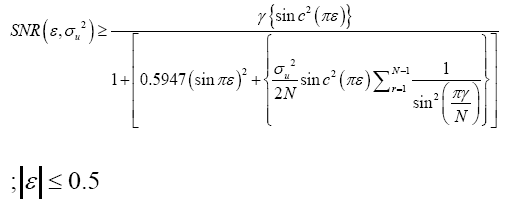(7)

This Signal to Interference plus Noise Ratio or SINR is in terms of CFO and the variance of σ2. This is without timing jitter (ξ). The equation (8) for SNR is including the timing jitter (ξ) is shown below,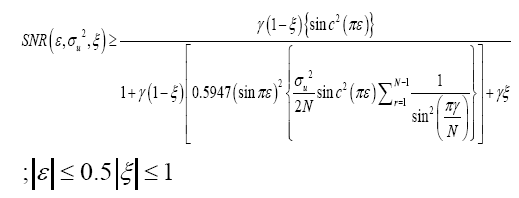(8)

Now the Bit Error Rate can be determined with the help of E0/N0 the Equation (9) below,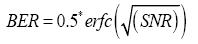(9)

Orthogonal frequency division multiplexing (OFDM) transforms a frequency selective channel into huge set of individual frequency non-selective narrowband channels that is fitted to a multiple-input multiple-output (MIMO) structure that needs a frequency nonselective characteristic at every channel once the transmission rate is high enough to form the entire channel frequency selective. Therefore, a MIMO system using OFDM, denoted MIMO-OFDM, is ready to realize high spectral efficiency. However, the adoption of multiple antenna parts at the transmitter for spatial transmission outcomes a superposition of multiple transmitted signals at the receiver weighted by their corresponding multipath channels and makes the reception tougher. This imposes a true challenge on the way to design a sensible system which will supply a real spectral efficiency improvement. If the channel is frequency selective, the received signals are distorted by ISI that makes the detection of transmitted signals troublesome. OFDM has emerged jointly of best ways that to get rid of such ISI [8,9].

Space time block code

In this paper the performances have been shown in non-STBC OFDM system and with STBC OFDM. The performance of both the system has been derived in equation of Signal to Noise Ratio (SNR) and Bit Error Rate (BER) for 2:1 transmission system. This paper extended the work by deriving the equations for 4:1 transmission system. After that performances have been shown by plotting various graphs. Results also show the effect of the Inter Carrier Interference (ICI), Channel Estimator Error, and the Additive White Gaussian Noise (AWGN). Mapping is used to calculate the BER and equations for the SNR and BER have been derived analytically.

System model for STBC-OFDM

We consider an OFDM system with transmit diversity, in which the total system bandwidth is divided into N equally spaced and orthogonal sub-carriers. We investigate the system with four transmission antennas and one receiving antenna. During the first time instant, the four symbols [X0 X1 X2 X3] are transmitted from four antennas simultaneously, with X0, X1, X2 and X3 transmitted from all four antennas. In the second time slot [-X1* X0* -X3* X2], third time slot [-X2* -X3* X0* X*] and fourth time slot [X3 -X2 -X1 X0] are transmitted. This encoding of the transmitted symbol sequence from the transmit antennas is given by then encoding matrix Equation (10),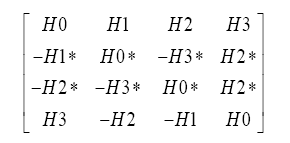(10)

For each transmit antenna, a block of N complex-valued data symbols {X(k)} for k=0 to N-1 are grouped and converted into a parallel set to form the input to the OFDM modulator, where k is the sub carrier index and N is the number of sub carriers. The modulator consists of an Inverse Fast Fourier transform (IFFT) block. The output of the IFFT at each transmitter is the complex baseband modulated OFDM symbol in discrete time domain and is given by equation (11),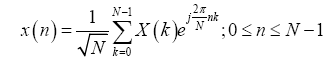(11)

The channel is modeled by a tapped delay line with channel coefficients that are assumed to be slowly varying such that they are almost constant over the two transmission instants. The channel frequency response for the kth subcarrier is Where h(p) is the complex channel gain of the pth multipath component.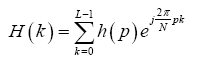(12)

Where h(p) is the complex channel gain of the pth multipath component. The time-domain received signals at the first and second transmission instances at the input to the FFT block are respectively given by Equations (13)-(16)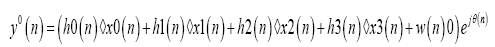(13)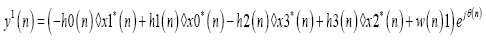(14)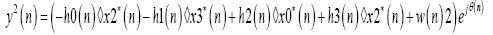(15)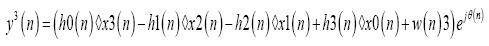(16)

Where ◊ represents linear convolution, subscripts indicate antenna index, and superscripts indicate transmission instant. The complex Gaussian random variable w(n) represents the Additive White Gaussian Noise (AWGN) term with ζ w 2= E[lw(n))|2], and θ(n) is the phase noise. Detection with Imperfect Channel Estimation: In the presence of imperfect channel estimation, we assume a channel estimation model such that the channel estimates H of the true channel H is given equation (17)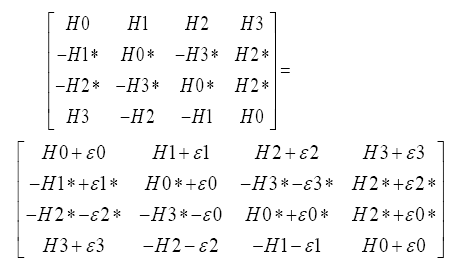(17)

Where ε0, ε1, ε2 and ε3 are the errors in the channel estimate from the first, second, third and fourth transmit antennas respectively, and are modeled as independent zero-mean complex Gaussian random variables with variances 2σε02, 2σε1 2, 2σε2 2, 2σε3 2 respectively.

Variance: As the noise signal has both positive and negative amplitude, it is squared and then the mean has been taken, which is variance. We consider the variance of noise for calculation. The variance of the noise W, after some mathematical manipulations, is given by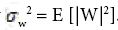.

Calculation of SNR and BER: The bit error rate for the case of 16QAM modulation using Gray code mapping for (b1b2b3b4). It is important to note that although the presentation is only for 16QAM, the following analysis is valid for all square QAM constellations. The conditional BER for bit b1, condition on H0, H1, H2, and H3 is given by Equation (18),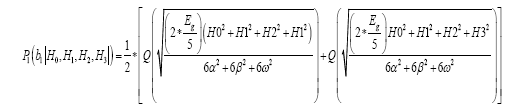(18)

and for bit b3 is given by Equation (19)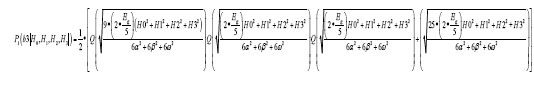(19)

From which the SNR γ is given by Equation (20),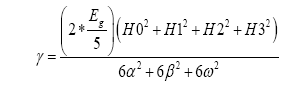(20)

Probability density function given by Equation (21),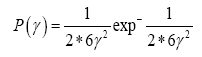(21)

Due to the symmetry of square M-QAM constellations, the BER for the in-phase and quadrature bits are equal such that Pe (b1) = Pe (b2) and Pe (b3)= Pe (b4 ). Therefore the average BER is obtained by averaging the conditional BER of b1 and b3 over the PDF of the SNR γ. The average BER is therefore given by equation (22),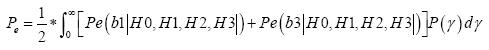(22)

#### Results

The Figure 1 shows the graph between SNR vs. normalized CFO where x-axis denotes the normalized CFO and y-axis denotes the SNR (dB). It is observed that when CFO is zero then SNR is high. And after that SNR is exponentially decreasing with the increasing of CFO. Graph shows the four plots for different value of variance of the phase noise. Figure 2 shows the graph between SNR vs. timing jitter. It is observed that if the delay or the timing jitter increases then the signal strength decreased. For this the SNR is also decreasing. There are plotting shows different graphs for different values of phase noise and CFO. Figure 3 shows the SNR vs. BER graph at different value of noise. From the graph it has been seen that for v=0.3, we get highest SNR curve. And for this highest SNR value the BER is decreasing fast compared to the other value of phase value v and corresponding SNR. Figure 4 show the graph of SNR vs. BER for 4:1 transmission system along with 2:1 and 1:1 system. It is clearly seen that 4:1 graph is closer to the two axes than the others. It means that the BER is decreasing fast when the number of antennas increases. It shows that transmission power decreases when number of antennas increase. Another analysis can be drained from the graph shown is that or fixed value of transmission power the noise term can be reduced by increasing the number of antennas.

#### Conclusion

In this paper the equations for SNR and BER using 2:1 and 4:1 transmission system are derived. The result obtained with good accuracy. The effects of phase noise, timing jitter and carrier interference on the system are analyzed. The SNR vs. BER curve shows that increasing the diversity gain improve the performance of the system.

#### References

Select your language of interest to view the total content in your interested language

### Article Usage

• Total views: 10061
• [From(publication date):
September-2016 - Dec 13, 2019]
• Breakdown by view type
• HTML page views : 9900Can't read the image? click here to refresh